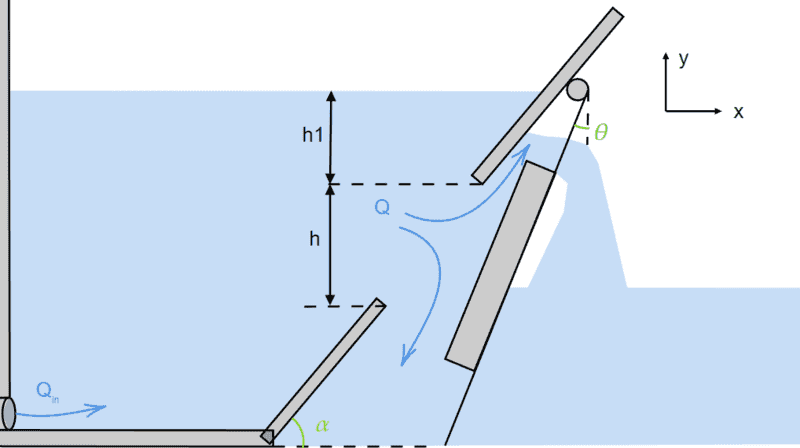# Flow through hinged hatch on inclined wall

• Engineering
• Kapper

#### Kapper

Homework Statement
I have a tank, where I want to determine the outflow through an orifice. The problem is that the orifice opening is controlled by a hatch with varying angles dependent on the sum of torques.
Relevant Equations
Orifice equationSo far I have only been able to come up with an equation for the flow (Q) using the orifice equation through the inclined area and thus not dependent on the angle.

Can someone help me with an expression for this?

Would not the flow out the bottom be the adjacent side of triangle with angle alpha? You could use a trig ratio to express that bottom side.